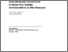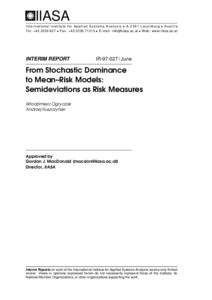# From Stochastic Dominance to Mean-Risk Models: Semideviations as Risk Measures

Ogryczak, W. & Ruszczynski, A. (1997). From Stochastic Dominance to Mean-Risk Models: Semideviations as Risk Measures. IIASA Interim Report. IIASA, Laxenburg, Austria: IR-97-027Preview
Text
IR-97-027.pdf

## Abstract

Two methods are frequently used for modeling the choice among uncertain outcomes: stochastic dominance and mean-risk approaches. The former is based on an axiomatic model of risk-averse preferences but does not provide a convenient computational recipe. The latter quantifies the problem in a lucid form of two criteria with possible trade-off analysis, but cannot model all risk-averse preferences. In particular, if variance is used as a measure of risk, the resulting mean-variance (Markowitz) model is, in general, not consistent with stochastic dominance rules. This paper shows that the standard semideviation (square root of the semivariance) as the risk measure makes the mean-risk model consistent with the second degree stochastic dominance, provided that the trade-off coefficient is bounded by a certain constant. Similar results are obtained for the absolute semideviation, and for the absolute and standard deviations in the case of symmetric or bounded distributions. In the analysis we use a new tool, the Outcome-Risk diagram, which appears to be particularly useful for comparing uncertain outcomes.

Item Type: Monograph (IIASA Interim Report) Risk, Modeling, Policy (RMP) IIASA Import 15 Jan 2016 02:09 27 Aug 2021 17:16 http://pure.iiasa.ac.at/5264View Item